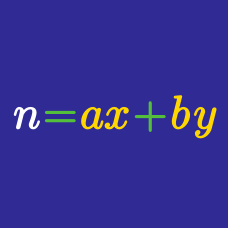Number Theory

# Integer Equations - With Restriction

Ben throws a fair die five times. If we label the outcome of the $$n^{\text{th}}$$ throw as $$a_n,$$ what is the number of cases such that $$a_1< a_2<a_3\le a_4\le a_5?$$

Consider the two sets $$A=\{1,2,3,4,5,6\}$$ and $$B=\{7,8,9,10\}.$$ How many functions $$f:A\rightarrow B$$ are there that satisfy $$f(1)\le f(2)\le f(3)<f(4)\le f(5)\le f(6)?$$

How many 4-digit numbers are there such that the digit sum is $$9?$$

How many 4-digit numbers are there such that the digit sum is $$11?$$

Ben throws a fair die six times. If we label the outcome of the $$n^{\text{th}}$$ throw as $$a_n,$$ what is the number of cases such that $$a_1\le a_2<a_3\le a_4\le a_5\le a_6?$$

×